1588560780

# Understanding K-Means Clustering Algorithm

## What Is K-means Clustering?

When trying to analyze data, one approach might be to look for meaningful groups or clusters. Clustering is dividing data into groups based on similarity. And K-means is one of the most commonly used methods in clustering. Why? The main reason is its simplicity.

In this tutorial, we’ll start with the theoretical foundations of the K-means algorithm, we’ll discuss how it works and what pitfalls to avoid. Then, we’ll see a practical application of the K-means algorithm with Python using the sklearn library.

So, let’s get right into it and see what K-means is all about.

## How Does K-means Clustering Work?

Let’s say we’d like to divide the following points into clusters.First, we must choose how many clusters we’d like to have. The K in ‘K-means’ stands for the number of clusters we’re trying to identify. In fact, that’s where this method gets its name from. We can start by choosing two clusters.

The second step is to specify the cluster seeds. A seed is basically a starting cluster centroid. It is chosen at random or is specified by the data scientist based on prior knowledge about the data.

One of the clusters will be the green cluster, and the other one – the orange cluster. And these are the seeds.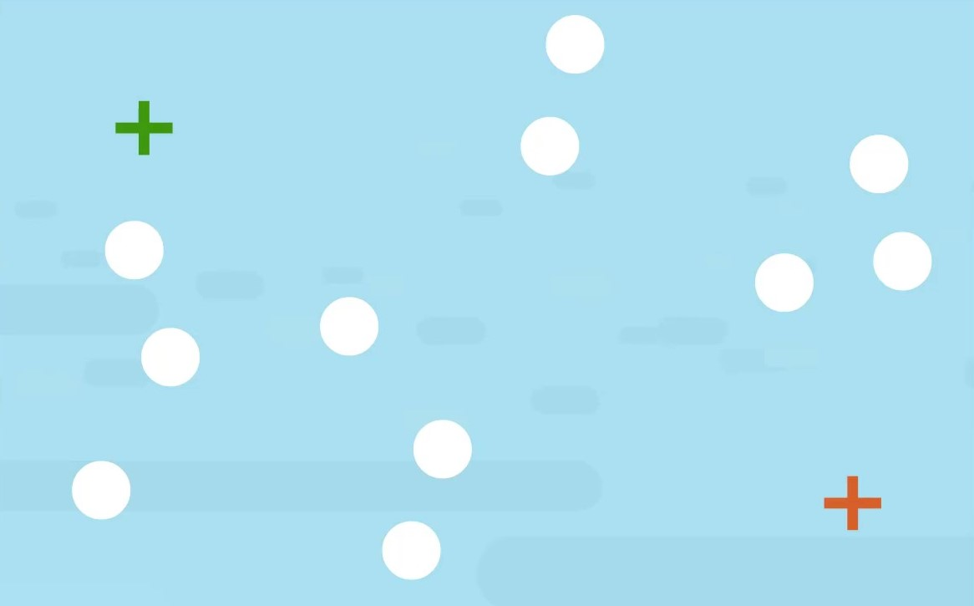The next step is to assign each point on the graph to a seed. Which is done based on proximity.

For instance, this point is closer to the green seed than to the orange one. Therefore, it will belong to the green cluster.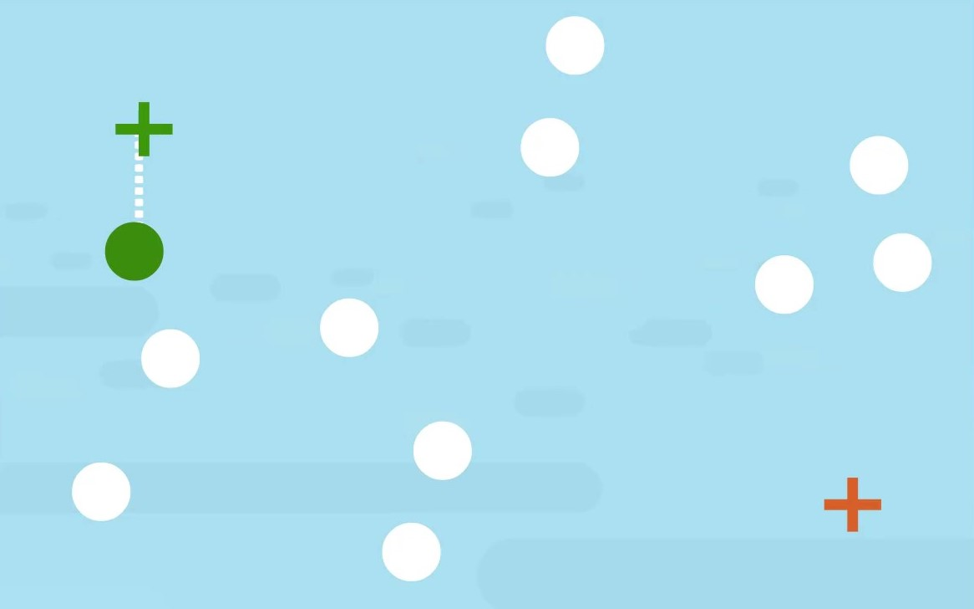This point, on the other hand, is closer to the orange seed, therefore, it will be a part of the orange cluster.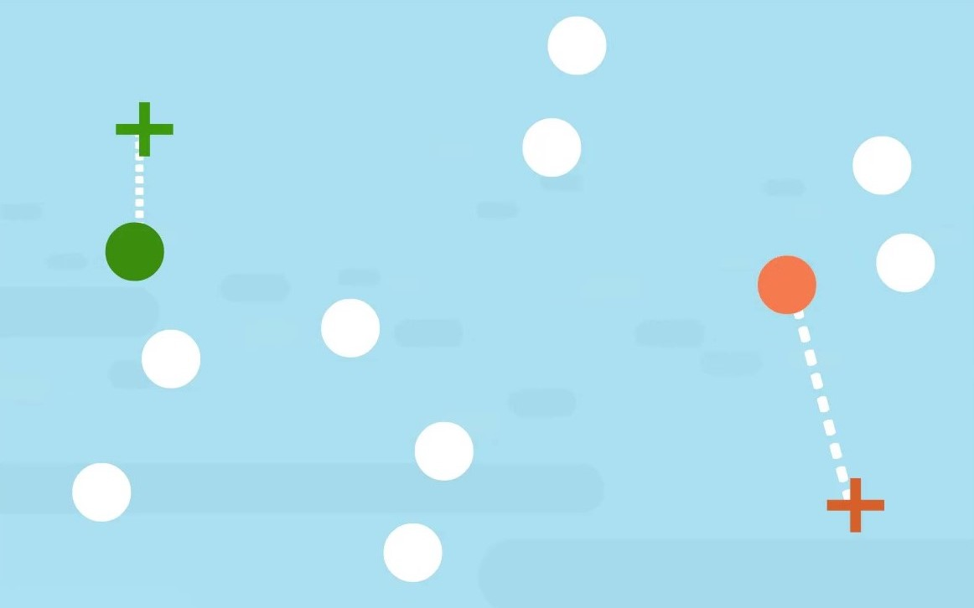In this way, we can assign all points on the graph to a cluster, based on their Euclidean squared distance from the seeds.

The final step is to calculate the centroid or the geometrical center of the green points and the orange points. The green seed will move closer to the green points to become their centroid and the orange will do the same for the orange points.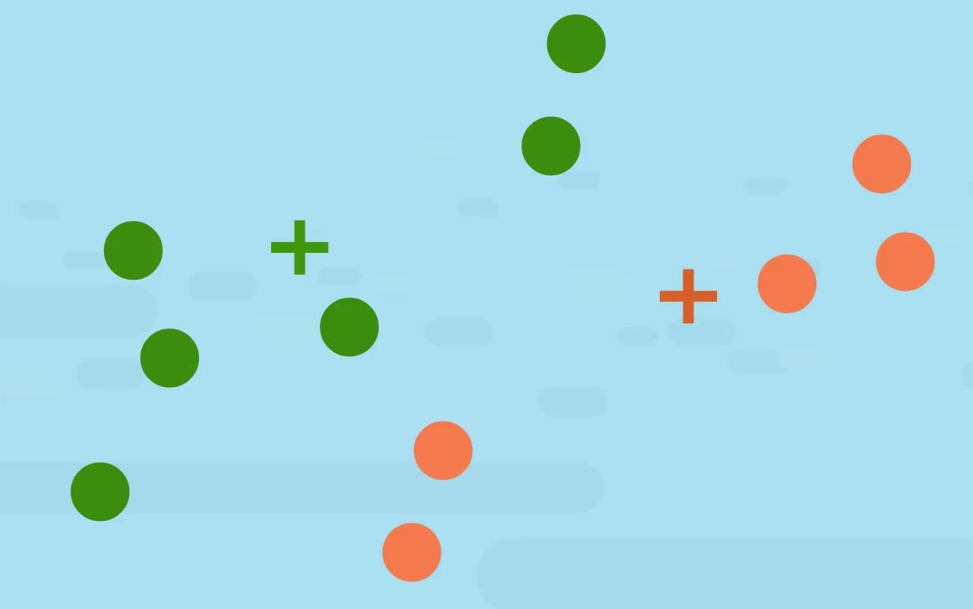From here, we can repeat the last two steps. We can do that 10, 15 or 1000 times until we’ve reached a clustering solution where we can no longer adjust any of the clusters.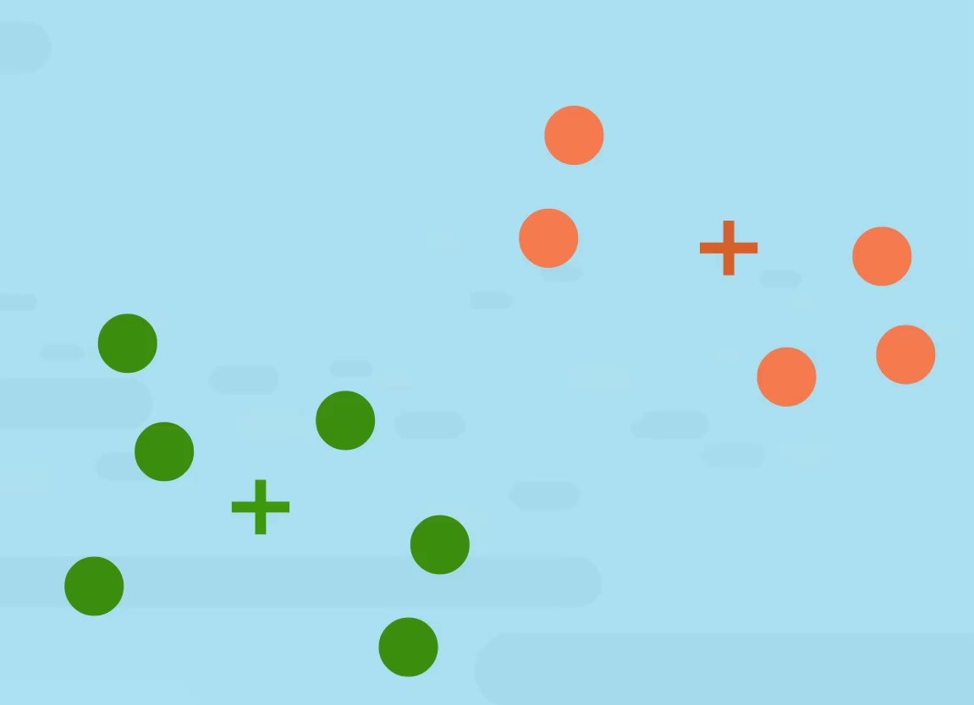Sounds simple, right?

However, due to its simplicity, K-means is prone to some issues.

## What Are the Disadvantages of K-means?

One disadvantage arises from the fact that in K-means we have to specify the number of clusters before starting. In fact, this is an issue that a lot of the clustering algorithms share. In the case of K-means if we choose K too small, the cluster centroid will not lie inside the clusters.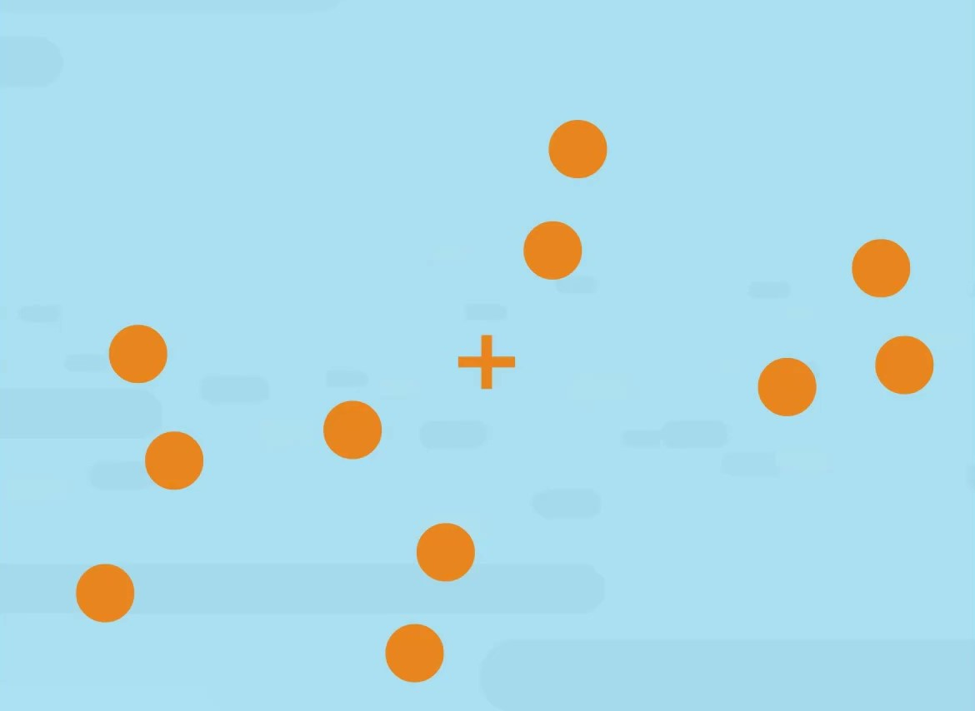As we can see, in this example, this is not representative of the data. In cases where K is too large, some of the clusters may be split into two. Reasonable enough, right?

Another important issue is that K-means enforces clusters with a spherical shape or blobs. The reason is that we are trying to minimize the distance from the centroids in a straight line. So, if we have clusters, which are more elongated, K-means will have difficulty separating them.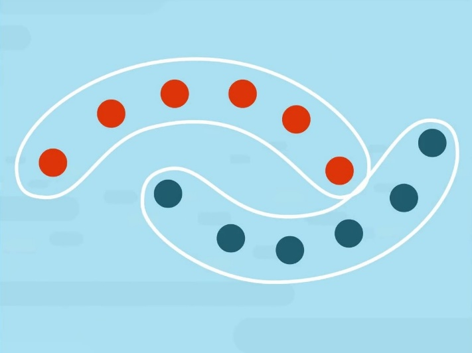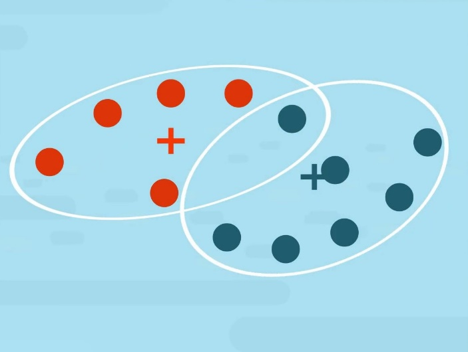Now that you’re familiar with what K-means is and how it works, let’s bring theory into practice with an example.

## What’s K-Means Clustering’s Application?

One of K-means’ most important applications is dividing a data set into clusters. So, as an example, we’ll see how we can implement K-means in Python. To do that, we’ll use the sklearn library, which contains a number of clustering modules, including one for K-means.

Let’s say we have our segmentation data in a csv file. After we’ve read the file (in our case using the pandas method) we can proceed with implementing K-means.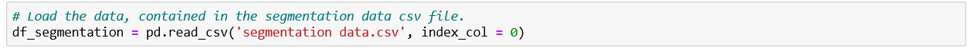You’ll need to import ‘KMeans’ from ‘sklearn cluster’.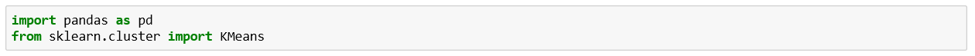As mentioned above, K-means doesn’t tell us how many clusters there are. Instead, it minimizes the Euclidean norm.

### However, we usually want to be able to determine the number of clusters, too, right?

Well, what if we assume there are 2 clusters and we calculate the sum of squared distances within each of the clusters? We can then assume we’ve got 3 clusters and do the same. Then we can compare the two and see how drastic the change is.

In reality, this is exactly what is done. Usually, we run the algorithm, say, 10 different times with 10 different number of clusters. We calculate the Within Cluster Sum of Squares or ‘W C S S’ for each of the clustering solutions. The WCSS is the sum of the variance between the observations in each cluster. It measures the distance between each observation and the centroid and calculates the squared difference between the two. Hence the name: within cluster sum of squares.

So, here’s how we use Within Cluster Sum of Squares values to determine the best clustering solution.First, we need to initialize the Within Cluster Sum of Squares variable. It will begin life as an empty list. But there are worse faiths, I’m sure. Then we’ll run a for loop, trying out several clustering solutions, calculating their WCSS and adding the value to this list. The next step is determining the size of the loop.

## How Many Iterations Do We Run?

Let’s run the code with 10 iterations. So, a range from one to eleven. You can try running the algorithm with a larger number. In this particular example, it wouldn’t improve the results, so, we’ll stick with 10. However, depending on the data you may need to increase the number of iterations.

In the loop, we run the K-means method. We set the number of clusters to ‘i’ and initialize with ‘K-means ++’. K-means ++ is an algorithm which runs before the actual k-means and finds the best starting points for the centroids.

The next item on the agenda is setting a random state. This ensures we’ll get the same initial centroids if we run the code multiple times.

Then, we fit the K-means clustering model using our standardized data. The statement fits a K-means clustering model with ‘i’ clusters to it.

And lastly, in each iteration, we add a value to the WCSS array. The WCSS is stored in the inertia attribute.

## How to Choose the Number of Clusters?

The next step is to plot the results. We’ll plot ‘W C S S’ values against the number of clusters.

Here is the relevant code: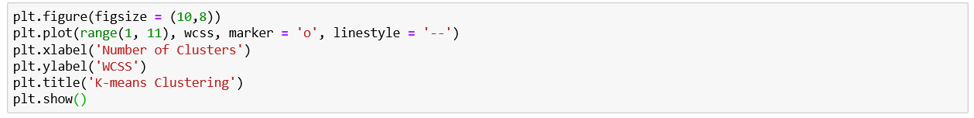On the x-axis, we will have the range from 1 to 11. On the y-axis, we will have the WCSS variable.

You can also set a marker and line style for some added visual appeal. It’s up to you. And you can see in the picture bellow the result from executing the code: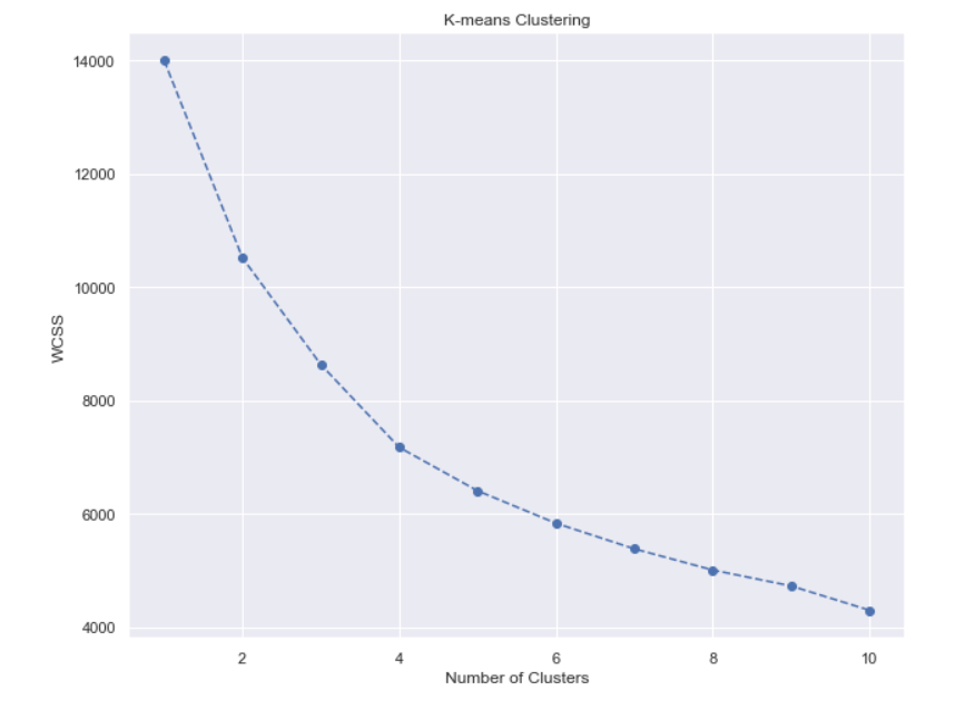We have our plot, so let’s analyze it.

We see the function is monotonically decreasing. Sometimes it can be rapidly declining, other times – more smoothly. Depending on the shape of this graph, we make a decision about the number of clusters.

### But how exactly do we choose how many clusters we want?

We’ll use an approach known as the ‘Elbow method’. As you can see, this graph looks like an arm with an elbow. The goal here is to spot the elbow itself and take that many clusters. Usually, the part of the graph before the elbow would be steeply declining, while the part after it – much smoother.

It seems we’ve got a clear winner: the Elbow on the graph is at the 4-cluster mark. This is the only place until which the graph is steeply declining, while smoothening out afterwards.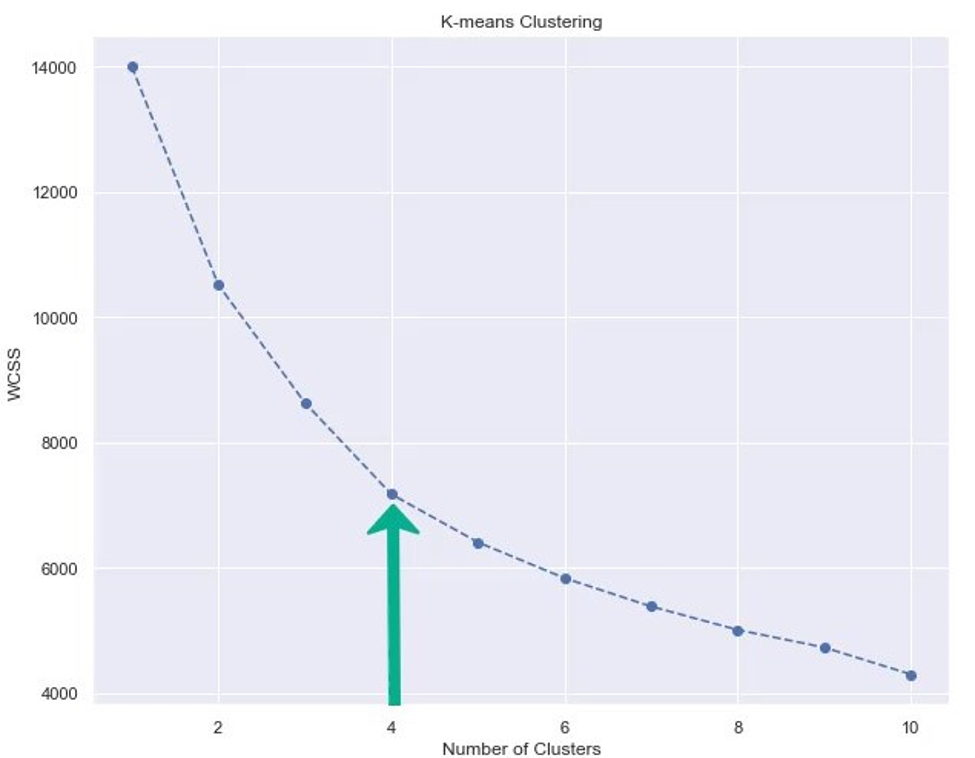Note here, that because we have only four clusters, the algorithm with more than 10 will not yield an improvement in the results. But in cases where there are more clusters, a loop with more iterations should be performed.

Now we can perform K-means clustering with 4 clusters.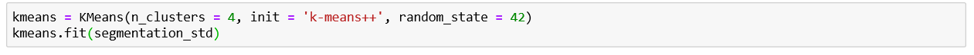We initialize with K-means ++ again and we’ll use the same random state: 42. Finally, we must fit the data.

And that’s all you need to perform K-means Clustering in Python.

Hopefully, we shed some light on how K-means works and how to implement it in Python.

Original article source at https://365datascience.com

#machinelearning #python #datascience #artificialintelligence #kmeans

## Buddha Community1621443060

## Understanding Core Data Science Algorithms: K-Means and K-Medoids Clustering

This article provides an overview of core data science algorithms used in statistical data analysis, specifically k-means and k-medoids clustering.

Clustering is one of the major techniques used for statistical data analysis.

As the term suggests, “clustering” is defined as the process of gathering similar objects into different groups or distribution of datasets into subsets with a defined distance measure.

K-means clustering is touted as a foundational algorithm every data scientist ought to have in their toolbox. The popularity of the algorithm in the data science industry is due to its extraordinary features:

• Simplicity
• Speed
• Efficiency

#big data #big data analytics #k-means clustering #big data algorithms #k-means #data science algorithms1624333080

## K-Means Clustering Algorithm

### Clustering

K-means is one of the simplest unsupervised machine learning algorithms that solve the well-known data clustering problem. Clustering is one of the most common data analysis tasks used to get an intuition about data structure. It is defined as finding the subgroups in the data such that each data points in different clusters are very different. We are trying to find the homogeneous subgroups within the data. Each group’s data points are similarly based on similarity metrics like a Euclidean-based distance or correlation-based distance.

The algorithm can do clustering analysis based on features or samples. We try to find the subcategory of sampling based on attributes or try to find the subcategory of parts based on samples. The practical applications of such a procedure are many: the best use of clustering in amazon and Netflix recommended system, given a medical image of a group of cells, a clustering algorithm could aid in identifying the centers of the cells; looking at the GPS data of a user’s mobile device, their more frequently visited locations within a certain radius can be revealed; for any set of unlabeled observations, clustering helps establish the existence of some structure of data that might indicate that the data is separable.

## What is K-Means Clustering?

K-means the clustering algorithm whose primary goal is to group similar elements or data points into a cluster.

K in k-means represents the number of clusters.

A cluster refers to a collection of data points aggregated together because of certain similarities.

K-means clustering is an iterative algorithm that starts with k random numbers used as mean values to define clusters. Data points belong to the group represented by the mean value to which they are closest. This mean value co-ordinates called the centroid.

Iteratively, the mean value of each cluster’s data points is computed, and the new mean values are used to restart the process till the mean stops changing. The disadvantage of k-means is that it a local search procedure and could miss global patterns.

The k initial centroids can be randomly selected. Another approach of determining k is to compute the entire dataset’s mean and add _k _random co-ordinates to it to make k initial points. Another method is to determine the principal component of the data and divide it into _k _equal partitions. The mean of each section can be used as initial centroids.

#data-science #algorithms #clustering #k-means #machine-learning1600190040

## SciPy Cluster - K-Means Clustering and Hierarchical Clustering

SciPy is the most efficient open-source library in python. The main purpose is to compute mathematical and scientific problems. There are many sub-packages in SciPy which further increases its functionality. This is a very important package for data interpretation. We can segregate clusters from the data set. We can perform clustering using a single or multi-cluster. Initially, we generate the data set. Then we perform clustering on the data set. Let us learn more SciPy Clusters.

#### K-means Clustering

It is a method that can employ to determine clusters and their center. We can use this process on the raw data set. We can define a cluster when the points inside the cluster have the minimum distance when we compare it to points outside the cluster. The k-means method operates in two steps, given an initial set of k-centers,

• We define the cluster data points for the given cluster center. The points are such that they are closer to the cluster center than any other center.
• We then calculate the mean for all the data points. The mean value then becomes the new cluster center.

The process iterates until the center value becomes constant. We then fix and assign the center value. The implementation of this process is very accurate using the SciPy library.

#numpy tutorials #clustering in scipy #k-means clustering in scipy #scipy clusters #numpy1601196420

## Master KMeans clustering basics

### Types of Clustering:

Clustering comes under the data mining topic and there is a lot of research going on in this field and there exist many clustering algorithms.

The following are the main types of clustering algorithms.

1. K-Means
2. Hierarchical clustering
3. DBSCAN

### Applications of Clustering:

Following are some of the applications of clustering

1. Customer Segmentation: This is one of the most important use-cases of clustering in the sales and marketing domain. Here the aim is to group people or customers based on some similarities so that they can come up with different action items for the people in different groups. One example could be, amazon giving different offers to different people based on their buying patterns.
2. Image Segmentation: Clustering is used in image segmentation where similar image pixels are grouped together. Pixels of different objects in the image are grouped together.

#machine-learning #k-means-clustering #clustering #k-means1595334123

## Authentication In MEAN Stack - A Quick GuideI consider myself an active StackOverflow user, despite my activity tends to vary depending on my daily workload. I enjoy answering questions with angular tag and I always try to create some working example to prove correctness of my answers.

To create angular demo I usually use either plunker or stackblitz or even jsfiddle. I like all of them but when I run into some errors I want to have a little bit more usable tool to undestand what’s going on.

Many people who ask questions on stackoverflow don’t want to isolate the problem and prepare minimal reproduction so they usually post all code to their questions on SO. They also tend to be not accurate and make a lot of mistakes in template syntax. To not waste a lot of time investigating where the error comes from I tried to create a tool that will help me to quickly find what causes the problem.

``````Angular demo runner
Online angular editor for building demo.
ng-run.com
<>
``````

Let me show what I mean…### Template parser errors#

There are template parser errors that can be easy catched by stackblitzIt gives me some information but I want the error to be highlighted#mean stack #angular 6 passport authentication #authentication in mean stack #full stack authentication #mean stack example application #mean stack login and registration angular 8 #mean stack login and registration angular 9 #mean stack tutorial #mean stack tutorial 2019 #passport.js